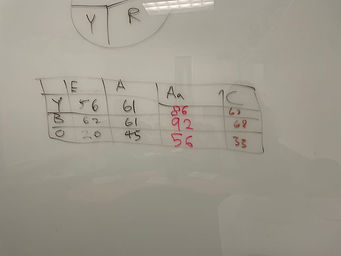## Mr. Jake

### Target 1​

###### Lesson Type:

Continuation

Data Probability

:

Interpreting Data

Construct and read graphs from given information to solve problems.

###### 1:

Understand that graphs visually illustrate data and help make sense of data quickly.

###### 2:

Understand that a graph helps the reader visualize the information (see the data).

###### 3:

Understand that graphs are used to communicate detailed information clearly and concisely.

###### 4:

Accurately use the vocabulary associated with graphs.

6th

###### Vocabulary:

Stacked Bar Graph, Range

Activities:

Collected data to create stacked bar graphs

Answered questions using data from stacked bar graphs### Home Exploration

###### Guiding Questions:## Absent Students:

### Target 2

:

###### 1:

Find the area of triangles, trapezoids, and parallelograms.

###### 2:

Use previous area understandings (of rectangular figures) to gain insight into the area of new geometric shapes.

###### 3:

Determine the algebraic equations for calculating the area of a triangle (½ base * height), a trapezoid (½ height * (base1 + base2)), and a parallelogram (base * height).

###### 4:

Calculate the area of a shaded region of a figure.

7th

###### Vocabulary:

Area, Triangle, Formula

Activities:

Discussed how to find the area of a triangle

Derived the equation for area of a triangle using knowledge of area of rectangles

Found the area of the shaded region of a figure### Home Exploration

###### Guiding Questions:### Target 3

:

###### Vocabulary:

Activities:### Home Exploration